# Circumference and Area of CirclesIn this lesson, you will extend your knowledge of perimeter and area to include circles. You will also learn to write and solve problems that involve comparison between the circumference and area of circles, using both the radius and diameter.

For a polygon, the distance around the figure is called the perimeter. For a circle, the distance around the figure is called the circumference of the circle. The diameter is the distance across the circle through its center. The radius is the distance from the center to any point on the circle and is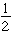the size of the diameter.

The ratio of the circumference to the diameteris the same for every circle and is represented by the Greek letter,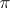, known as pi. We approximate the decimal for pi as 3.14 and use the fraction,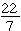.

Remember that the diameter is twice the radius, so if you are given the radius, you can use this following formula: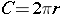### ExampleJeff is building a go-cart. The wheels he uses on the go-cart have a radius of 8 inches. What is the circumference of each wheel?

1. What is the formula for the circumference of a circle when the radius is given?C = 2πr
2. Use 3.14 for π and substitute the length of the radius into the formula.
C = 2(π)(8)
3. Multiply.
C = 2 x 3.14 x 8 = 50.24
The circumference of each wheel is about 50.24 inches.

### ExampleLet’s apply this knowledge to the following problem:

A circular swimming pool has a radius of 15 feet. The family that owns the pool is putting up a circular fence that is 5 feet away from the pool at all points. Approximately how many feet of fencing will they need for this project?

Before you start on the math, look at the dimensions that will be used to build the fence. We know the pool has a radius of 15 feet, but we will need to add 5 feet to that because the fence will be 5 feet away from the pool. Therefore, we will change the radius to 20 feet. Work this one out yourself.

1. What is the formula for the circumference of a circle when the radius is given?
2. Use 3.14 forand substitute the length of the radius into the formula.
3. Multiply.
They will need to purchase about 126 feet of fence.

### ExampleOne more problem using the diameter!

At Jackie’s pizza, pizzas are sold by their diameter. Rhyan orders a 14-inch pizza. What is the circumference of her pizza?

1. What is the formula for the circumference of a circle when the diameter is given?
2. Use 3.14 for and substitute the length of the radius into the formula.
3. Multiply.
Her pizza will be 43.96 inches around!

Take a look at the video to see ways to find circumference.Now we are going to learn how to find the area of a circle. You can use what you already know about circles, and π to find the area of a circle. The formula for finding the area of a rectangle is A=bh. Substitute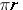for b and r for height.

Therefore, the formula for area of a circle: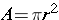Remember that the diameter is twice the radius, so if you are given the diameter, you must remember.

Careful! A common mistake is to multiply the radius by 2.
That is not the same as squaring the radius.

### ExampleThe face of a clock has a diameter of 12 inches. What is the area of the face of the clock?

1. How do you find the area of a circle when the diameter is given?
2. Use 3.14 for and substitute the radius into the formula.
3. Multiply.
The area of the face of the clock is about 113 square inches.

### Example

Let’s try one with the radius given – just plug in the numbers! Sara has a rose garden that is circular. The radius of the garden is 7 feet. What is the area of the garden?

1. How do you find the area of a circle when the radius is given?2. Use 3.14 for and substitute the radius into the formula.
3. Multiply.
The area of Sara’s rose garden is about 153.9 square feet.

Watch the Khan video below to see more examples of finding area of a circle.

ONE MORE THING…Sometimes, you may be asked to answer these questions in terms of.

A circular garden has an area of 64square yards. What is the circumference of the garden? Give your answer in terms of.

First, recall the formula for area of a circle:. Now, replace that with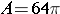. You can see that 64 replacedso find the square root of 64., oris 64. Now that we know the radius, we can find the diameter (2 x radius). So, the diameter is 16. If we answer in terms of, that means we leave the Greek letter and do not use 3.14.   The circumference is.

Formulas are Great!…If you know the formula, you simply plug in the known values to find the unknown values. I can use the area to find the radius, circumference, and diameter!

### Example: Area of a Circle is 50.24 square units*Use a calculator to find the square root.

To find the diameter: Find the radius and multiply by 2 diameter = 8

To find the circumference: 3.14 x 8 = 25.12

### Example: Circumference of a Circle is 69.08 units

To find the diameter: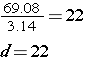To find the radius: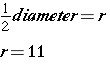To find the area:(source)